• F检验 对于多元线性回归模型，在对每个回归系数进行显著性检验之前，应该对回归模型的整体做显著性检验。这就是F检验。当检验被解释变量yt与一组解释变量x1, x2 , ... , xk -1是否存在回归关系时，给出的零假设...
F检验    对于多元线性回归模型，在对每个回归系数进行显著性检验之前，应该对回归模型的整体做显著性检验。这就是F检验。当检验被解释变量yt与一组解释变量x1, x2 , ... , xk -1是否存在回归关系时，给出的零假设与备择假设分别是
H0：b1 = b2 = ... = bk-1 = 0 ,
H1：bi, i = 1, ..., k -1不全为零。
首先要构造F统计量。由（3.36）式知总平方和（SST）可分解为回归平方和（SSR）与残差平方和（SSE）两部分。与这种分解相对应，相应自由度也可以被分解为两部分。
SST具有T - 1个自由度。这是因为在T个变差 ( yt -), t = 1, ..., T，中存在一个约束条件，即=
0。由于回归函数中含有k个参数，而这k个参数受一个约束条件制约，所以SSR具有k -1个自由度。因为SSE中含有T个残差，= yt -, t =
1, 2, ..., T，这些残差值被k个参数所约束，所以SSE具有T - k个自由度。与SST相对应，自由度T - 1也被分解为两部分，
(T -1) = ( k - 1) + (T - k)                                              (3.44)
平方和除以它相应的自由度称为均方。所以回归均方定义为
MSR = SSR / ( k - 1)
误差均方定义为
MSE = SSE / (T - k)
（显然MSE = s 2 (见3.23式)，它的期望是s 2）。定义F统计量为(3.45)
在H0成立条件下，有
F = ~ F(k -1, T - k)
设检验水平为 a ，则检验规则是
若用样本计算的F £ Fa (k -1, T - k)，则接受H0，
若用样本计算的F > Fa (k -1, T - k)，则拒绝H0。
拒绝H0意味着肯定有解释变量与yt存在回归关系。若F检验的结论是接受H0，则说明k – 1个解释变量都不与yt存在回归关系。此时，假设检验应该到此为止。当F检验的结论是拒绝H0时，应该进一步做t检验，从而确定模型中哪些是重要解释变量，哪些是非重要解释变量。
from：http://classroom.dufe.edu.cn/spsk/c102/wlkj/CourseContents/Chapter03/03_07_01.htm
http://classroom.dufe.edu.cn/spsk/c102/wlkj/CourseContents/Chapter03/

展开全文• 终于找到一篇全面而又简洁的讲多元线性回归模型检验方法的文章，涵盖了 主要的统计检验——F检验、t检验、DW检验
• 本课程为基于Python的数据分析和统计建模高级课程，针对多元线性回归模型的各种参数和统计量，通过Python代码手工复现，在代码复现的过程中结合数学公式和原理进行深入解读。这些统计量包括：回归系数参数估计、决定...最小二乘法 偏度与峰度
• 回归方程显著性检验——F检验3. 拟合优度评价指标I——R方/改进R方4. 拟合优度评价指标AIC/BIC/SIC写在最后 1. 单参数显著性检验——t检验 2. 回归方程显著性检验——F检验 3. 拟合优度评价指标I——R方/改进R方 4....
文章目录1. 前文回顾2. 单参数显著性检验——t检验2.1 问题的提出2.2 检验统计量——t统计量的构造2.3 拒绝域的构造2.4 浅谈p值3. 回归方程显著性检验——F检验3.1 问题的提出3.2 F检验统计量的构造3.3 拒绝域的构造4. 总结参考文献写在最后
【更新日志】
5/1/2020 对文章中公式与措辞中存在的问题进行修正(感谢评论区小伙伴的指正！)

1. 前文回顾
在上一篇文章中，我们分别研究了最小二乘估计量 β^OLS 和 σ^OLS 的相关性质，证明了 β^OLS 是 β 的一个最优线性无偏估计量（BLUE）， σ^2OLS  是 σ2 的一个无偏估计量，并得到了其在正态性误差假设下所对应的分布：
$\bm{\hat\beta}_{OLS} \thicksim N(\bm\beta, \sigma^2 ( \bm{X}^T \bm{X} )^{-1} )$$\frac {\hat \sigma _{OLS}^2} {\sigma^2} \thicksim \chi^2_{N-p-1}$
（详情请见：【统计学习系列】多元线性回归模型（三）——参数估计量的性质）。
通过最小二乘法拟合好模型的参数后，一个重要的问题就是：这个模型真的“好"吗？满足什么条件、什么性质的模型可以称作一个“好模型”呢？

2. 单参数显著性检验——t检验
2.1 问题的提出
首先，我们应该想到的问题是，在一个多元回归模型中，是不是每一个引入的自变量对因变量都有实实在在的影响呢？这样的影响是显著的吗？我们应不应该在模型中保留这一变量呢？
在回答这些问题之前，我们先回顾一下总体模型：
$Y= \beta_0 + \sum_{i=1}^{p} X_{i} \beta_i + \epsilon$其中：
$\epsilon \thicksim N(0,\sigma^2)$
让我们聚焦众多参数中的一个：βi 。βi 的意义是什么呢？当其他变量保持不变，而只有 Xi 变动时，每变动一个单位的 Xi，就会让 Y 平均变动 βi 个单位。而若 Xi 的变动能够确确实实引起 Y  的变动， 那么 βi 应该不等于0。换句话说，若可以验证 βi 不为0，那么就可以证明Xi 与 Y 存在线性相关关系。
【注1】 这里的关系是线性的。二次即更高阶的相关性并不能由 βi 是否等于0体现；
【注2】 Xi 与 Y 存在相关关系，并不能证明二者之间存在 因果关系（Causality）。
然而，我们现在只有 βi 的估计量 β^OLS,i ，而估计量与参数的真实值有一定的误差。由于 β^OLS,i 是一个统计量，因此只要我们在统计意义下验证 βi 是否等于零就可以了。
至此，我们就可以构造一个如下的假设检验问题：
$H_0: \beta_i=0 \\ H_1: \beta_i\ne0$
2.2 检验统计量——t统计量的构造
若想构造检验统计量，我们需要先对 β^OLS,i 进行变型。
记矩阵 (XTX)-1 的对角线元素：
$\text{diag}(\bm{X}^T \bm{X} )^{-1} = (v_{i,i})_{p+1}$
由第一部分中 β^OLS 服从的分布，我们可以得到 β^OLS,i 的分布：
$\hat\beta_{OLS, i} \thicksim N(\beta_i, \sigma^2 v_{i,i}) , \ i=0, 1,...,p$
将 β^OLS 标准化，有：
$\frac {\hat\beta_{OLS,i} - \beta_i}{ \sigma \sqrt{v_{i,i}} } \thicksim N(0, 1) , \ i=0, 1,...,p$
然而，此时总体标准差σ 为未知参数，因此需要用样本标准差 σ^ 来代替。由于 σ^2OLS 有分布：
$\frac {(N-P-1) \hat \sigma _{OLS}^2} {\sigma^2} \thicksim \chi^2_{N-p-1}$
由 t 分布的定义：
$\frac {\sqrt{(N-p-1)} (\hat\beta_{OLS,i} - \beta_i ) } { \sigma \sqrt{v_{i,i}} } / \sqrt{\frac {(N-p-1)\hat \sigma _{OLS}^2} {\sigma^2} } \\ =\frac {\hat\beta_{OLS,i} - \beta_i}{\hat \sigma \sqrt{v_{i,i}} } \thicksim t_{N-p-1}$
若原假设 H0 成立，即 βi = 0，可以定义 t 统计量（又称 t 值）：
$t= \frac {\hat\beta_{OLS,i}}{\hat \sigma \sqrt{v_{i,i}} } \thicksim t_{N-p-1}$
并称上式分母项为 β^OLS,i 的标准误（Standard Error, SE）。
从 t 统计量的定义式可以看出，t 的绝对值越大，β^OLS,i 越不等于0，原假设越有可能出错，我们越应该拒绝原假设。
注1：t 值的几何意义为 β^OLS,i 偏离其标准误的单位数；
注2：当N足够大时，t  统计量近似服从标准正态分布，因此可以使用标准正态分布进行替代。关于 t 分布与正态分布的关系，可参考文献 t分布收敛于标准正态分布的几种证明方法。
注3：关于t分布与t检验相关的更多知识，可参考文献 我懒得找了。
2.3 拒绝域的构造
然而，t 统计量多大算大呢？多大我们才应该拒绝原假设呢？
假设原假设 H0 正确，根据 t 统计量所对应的分布，在给定某一概率 1-α（我们称其为置信水平（Confidence Level））的前提下，t 统计量应该满足：
$P\{ |t| >t_{\frac{\alpha}{2}, N-p-1} \}< \alpha$
其中，tα/2,N-p-1是 tN-p-1 分布的 α/2 分位数，可以通过计算机软件或者查 t 分布分布表的方式得到。
在判断原假设是否成立时，我们可以基于一个原则：小概率事件在一次试验中几乎不会发生。若 α 足够小，比如规定为常用的0.05，那么在 H0 正确的前提下，t 统计量的绝对值大于 t0.025,N-p-1 的概率不超过5%。换言之，或者说 |t| > t0.025,N-p-1，我们就有足够的理由去认为原假设不正确，从而拒绝原假设。
基于这种思想，我们可以构造出一个区域（称为拒绝域（Rejection Field））：
$(-\infin,-t_{\frac{\alpha}{2}, N-p-1}) \ \cup \ (t_{\frac{\alpha}{2}, N-p-1}, +\infin)$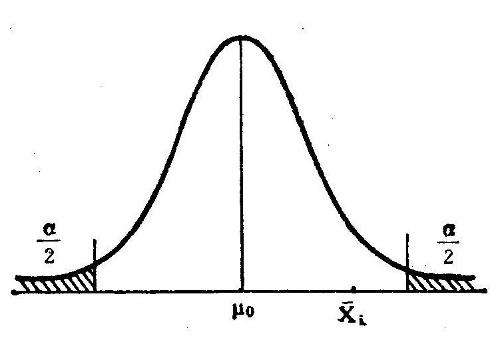图1 t检验拒绝域构造示意图（阴影部分即为对应显著性水平下的拒绝域。图片来源：百度图片）
当 t 统计量落入这个区域时，我们都应该拒绝原假设H0，并认为 βi 不等于0，自变量 Xi 与因变量 Y 存在统计意义下显著的线性相关关系（Statistically Significant Linear Correlation）。
注1：拒绝域，顾名思义，即若t值落入这个区间就应该拒绝原假设H0；
注2：在应用时，我们可以记住一句口诀：t值（的绝对值）越大越拒绝。
2.4 浅谈p值
此外，许多统计软件在回归的结果中会给出参数估计量所对应的p值（p-value）。p值的意义是：拒绝原假设所需要的最小置信度。什么意思呢？就是说，如果给出的p值小于你需要的置信度 α ，那么我们就应该拒绝原假设。也就是说，若：
$\text{p-value}<\alpha$
我们应拒绝原假设H0，并认为 βi 不等于0，自变量 Xi 与因变量 Y 存在统计意义下显著的线性相关关系。
注1：与t值正好相反，在应用时，我们可以说：p值越小越拒绝；
注2：使用p值而不使用t值的好处是：p值不依赖于样本容量 N，不用查分布表，使用起来更加简单方便 。

3. 回归方程显著性检验——F检验
3.1 问题的提出
在上一章的t检验中，我们对单一变量进行了显著性影响的评判。然而，这样做的一个缺点是：我们只判断了某一个解释变量对因变量单独的影响，而忽略了各解释变量对因变量的“共同作用”。若每一个变量单独与因变量不具有显著的线性关系，那么是不是模型本身就不能用了呢？未必。换句话说，只有模型中引入的解释变量 X1, …, Xp 均不能解释因变量Y，那么我们所建立的模型才能说是没有意义的。因此，我们需要对模型整体进行检验。与t检验类似，我们可以构造如下的假设检验问题：
$H_0: \beta_1 = \beta_2 = ... = \beta_p =0 \\ H_1: \exist \ i \in \{1,2,...,p\}, s.t. \ \beta_i\ne0$
注：β0 不能放入检验变量中，因为现在需要检验的是 X 与 Y 的线性关系，而不是Y 是否等于0这一问题。
3.2 F检验统计量的构造
我们应该如何构建检验统计量呢？让我们重新考察原模型：
$y_i= \beta_0 + \sum_{j=1}^{p} x_{ij} \beta_j + \epsilon_i$
然而，若原假设H0成立，那么模型将会退化为
$y = \beta_0 + \epsilon_i$
因此，假设回归方程是显著的（即H0不成立），那么由回归方程所拟合的因变量 y^i 应该有较大的方差，而其残差项所对应的方差应该较小，这是因为不同的解释变量 xi 应该对应不同的被解释变量  yi ；若回归方程不显著（H0成立），那么此时回归方程所拟合的变量 y^i 方差应该几乎为零，而其残差项 y^i - yi 则应具有较大的方差。
我们定义回归方程的可解释平方和（Explained Sum of Square，ESS）为：
$ESS = \sum_{i=1} ^N(\hat{y}_i - \bar{\hat{y}} )^2 = \sum_{i=1} ^N(\hat{y}_i - \bar{y} )^2$
回归的残差平方和（Residual Sum of Square，RSS）为：
$RSS = \sum_{i=1} ^N(\hat{y}_i - y_i )^2$
根据上述的分析，如果可解释平方（ESS）和与残差平方和（RSS）之比越大，则说明 X 对 Y 整体的影响越显著；若原假设H0成立，则ESS与RSS的比值应该接近于0。
而容易证明，ESS与RSS分别服从卡方分布：
$\frac{ESS}{p} \thicksim \chi^2_{p}$$\frac{RSS}{N-p-1} \thicksim \chi^2_{N-p-1}$
注：这部分证明将会在未来补充在附录中。
至此，我们可以构造F统计量：
$F = \frac{ESS/p}{RSS/N-p-1} \thicksim F(p, N-p-1)$
从F统计量的够造上来看，F统计量越大，说明 X 对 Y 存在的影响更大，原假设越可能被拒绝；而F统计量越接近零，说明 X 对 Y 存在的影响越小，原假设越可能成立。
注1：从F统计量的构造上来看，F取值非负（平方和与平方和的比值）；
注2：在应用过程中，可以记住口诀：F越大越拒绝；
注3：有关F分布与F检验更多的相关知识，请有关参考文献 我还是懒得找了。
3.3 拒绝域的构造
与 t 统计量拒绝域构造方法类似，在给定置信水平 1 - α 的前提下，F 统计量应该满足：
$P\{ F > F_{\alpha} (p, N-p-1) \}< \alpha$
其中，Fα(p, N - p - 1) 是 F(p, N - p - 1) 分布的 α 分位数，可以通过计算机软件或者查 F 分布分布表的方式得到。
注：与 t 统计量的双尾检验区间不同的是，F 检验为单尾检验。
我们依然依照“小概率事件在一次试验中不会发生”的原则：在 H0 正确的前提下，F 统计量大于 Fα(p, N - p - 1) 的概率不超过 α。换言之，若 F > Fα(p, N - p - 1)，我们就有足够的理由去认为原假设不正确，从而拒绝原假设。
基于这种思想，我们可以构造拒绝域：
$(F_\alpha(p, N-p-1), +\infin)$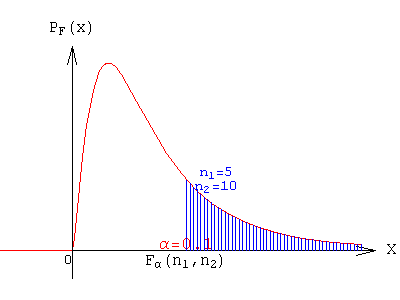图2 F检验拒绝域构造示意图（阴影部分即为对应显著性水平下的拒绝域。图片来源：百度图片）
当 F 统计量落入拒绝域内时，我们应该拒绝原假设H0，从而认为模型是显著的，或者说解释变量 X 与被解释变量 Y 之间存在显著的线性相关关系。

4. 总结
在这篇文章中，我们分别研究了单变量的显著性检验和模型的显著性检验。
（1）在单变量检验中，我们构造了假设检验问题：
$H_0: \beta_i=0 \\ H_1: \beta_i\ne0$
构造了检验统计量—— t 统计量：
$t= \frac {\hat\beta_{OLS,i}}{SE(\hat \beta_{OLS,i})}\thicksim t(N-p-1)$
并给出了拒绝域：
$(-\infin,-t_\frac{\alpha}{2}(N-p-1)) \ \cup \ (t_\frac{\alpha}{2}(N-p-1), +\infin)$
（2）在模型检验中，我们构造了假设检验问题：
$H_0: \beta_1 = \beta_2 = ... = \beta_p =0 \\ H_1: \exist \ i \in \{1,2,...,p\}, s.t. \ \beta_i\ne0$
构造了检验统计量—— F 统计量：
$F = \frac{ESS/p}{RSS/N-p-1} \thicksim F(p, N-p-1)$
并给出了拒绝域：
$(F_\alpha(p, N-p-1), +\infin)$
至此，我们解决了如何验证各个解释变量对因变量是否存在显著的线性影响，以及模型中涉及到的解释变量总体是否对因变量是否存在显著的线性影响。

参考文献
 t分布收敛于标准正态分布的几种证明方法

写在最后
欢迎感兴趣的小伙伴来跟作者一起挑刺儿~ 包括但不限于语言上的、排版上的和内容上的不足和疏漏~ 一起进步呀！
有任何问题，欢迎在本文下方留言，或者将问题发送至勘误邮箱： mikeysun_bugfix@163.com
谢谢大家！


展开全文• 为增加教学环节考试成绩评价与预测科学性,根据多元线性统计分析中的多元线性回归分析方法,对考试成绩指标进行量化,建立了考试成绩评价与预测的回归模型。解析国家四级英语考试成绩与学生期末考试成绩之间联系,...
• ## 多元线性回归检验

千次阅读 2020-07-13 10:43:33
多元线性回归模型通常用来研究一个应变量依赖多个自变量变化关系，如果二者以来关系可以用线性形式来刻画，则可以建立多元线性模型来进行分析。 1.t检验 t检验是对单个变量系数显著性检验，一般看p值；如果p...
多元线性回归模型通常用来研究一个应变量依赖多个自变量的变化关系，如果二者的以来关系可以用线性形式来刻画，则可以建立多元线性模型来进行分析。

1.t检验

t检验是对单个变量系数的显著性检验，一般看p值； 如果p值小于0.05表示该自变量对因变量解释性很强。

2.F检验

F检验是对整体回归方程显著性的检验，即所有变量对被解释变量的显著性检验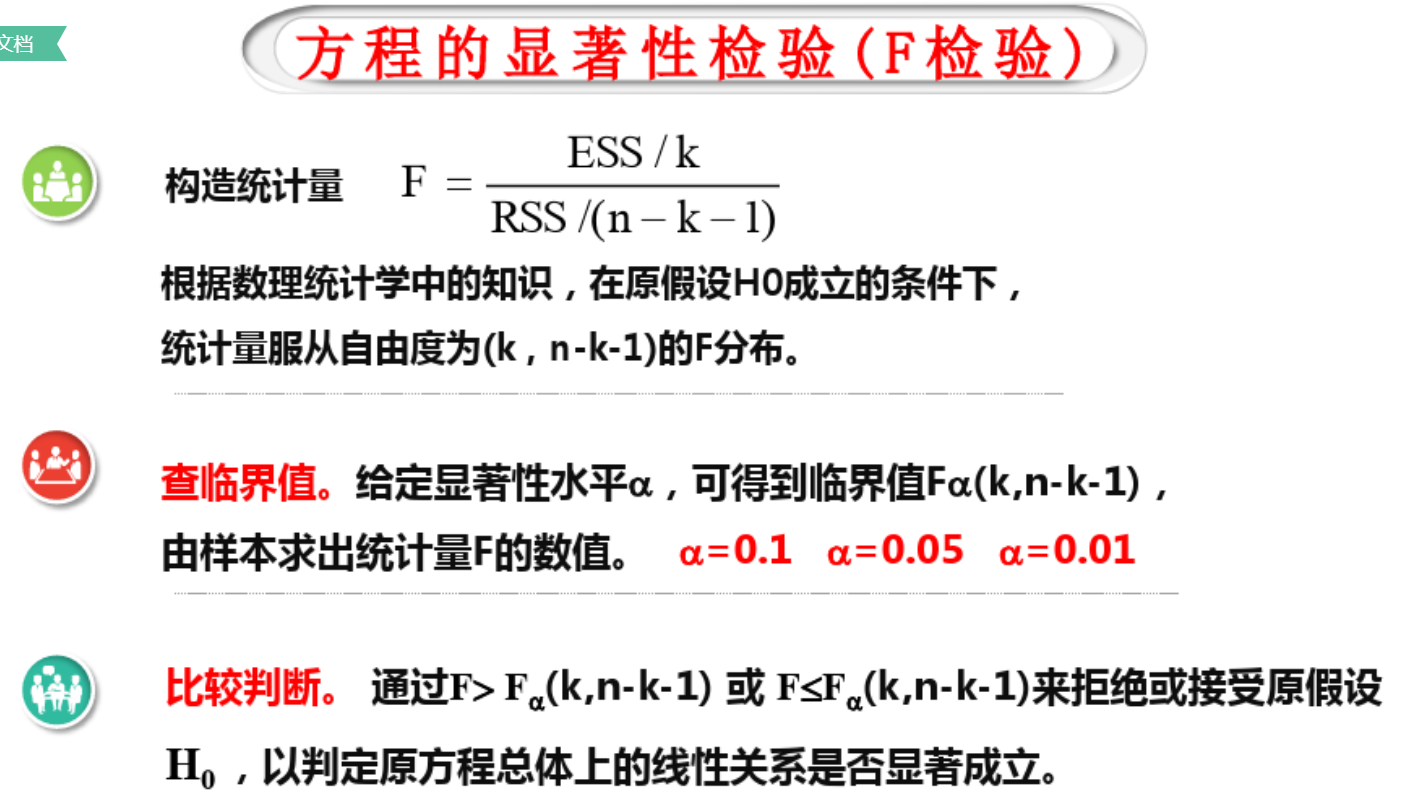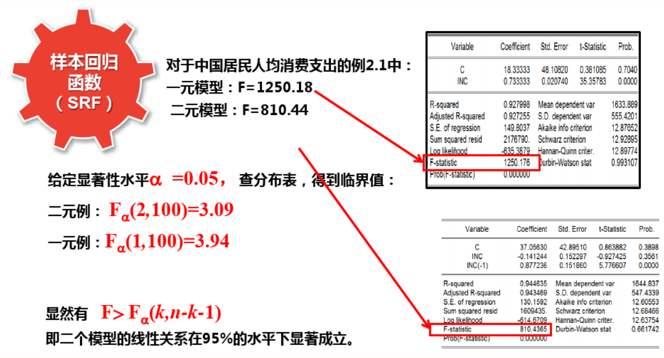F检验其通常是用来分析用了超过一个参数的统计模型，以判断该模型中的全部或一部分参数是否适合用来估计母体。

3.P值

P值就是t检验用于检测效果的一个衡量度，t检验值大于或者p值小于0.05就说明该变量前面的系数显著，选的这个变量是有效的。

4.R方

拟合优度检验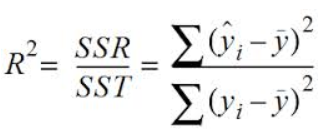R平方也有其局限性：R平方随着自变量的增加会变大，R平方和样本量是有关系的。因此，我们要到R平方进行修正。得到R平方值adjusted，来评判线性回归模型的拟合度。修正的方法：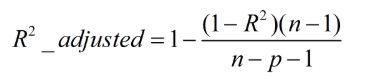n为样本数量，p为特征数量

消除了样本数量和特征数量的影响
5.调整后的R方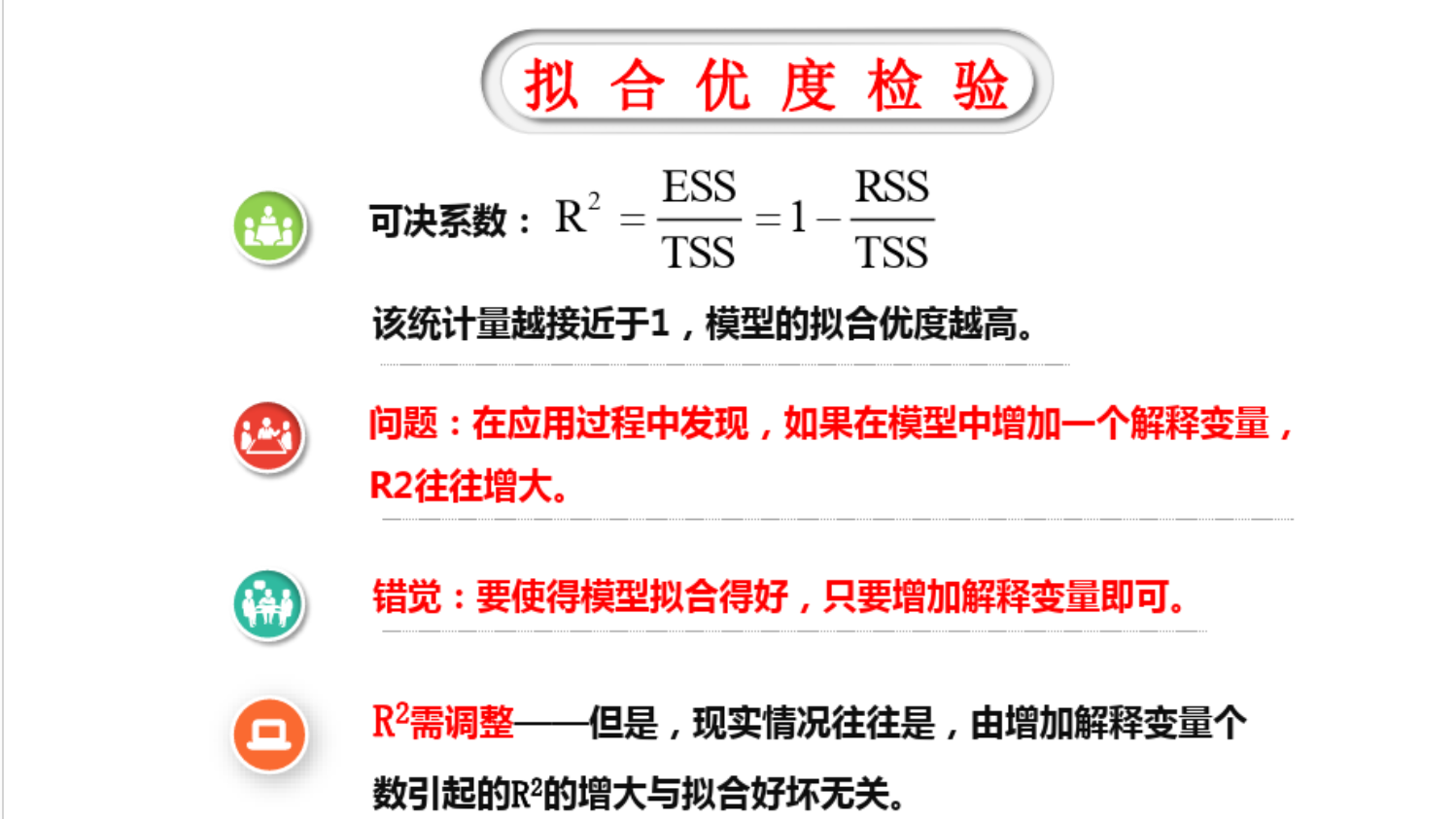t检验 --用于对各变量系数显著性检验 --判断标准：一般用p值 0.05来衡量  小于0.05 显著    大于0.05不显著

F检验 --整体回归方程显著性检验（所有自变量对因变量的整体解释） --判定：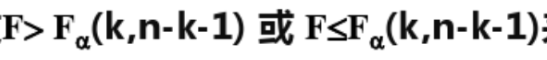需查统计分布表来确定

P值：就是用于t检验和F检验的衡量指标。

R方：整体回归方程拟合优度检验，R方的结果越接近于1越好，但是R方会因增加变量而增大，所以引进了调整R方检验。

调整R方：对R方检验的提升，避免受增加变量对R方的影响，配合向后删除模型观测。

不显著的原因概述：不显著有很多原因造成，可能是你的这个变量本身与被解释变量没有相关关系，所以不显著；也可能是解释变量过多，由多重共线性引起，也可能是其他原因。

在进行多元线性回归时，常用到的是F检验和t检验，F检验是用来检验整体方程系数是否显著异于零，如果F检验的p值小于0.05，就说明，整体回归是显著的。然后再看各个系数的显著性，也就是t检验，计量经济学中常用的显著性水平为0.05，如果t值大于2或p值小于0.05就说明该变量前面的系数显著不为0，选的这个变量是有用的。

https://www.cnblogs.com/tinglele527/p/12015449.html

展开全文• ##作业：分析影响中国人口自然增长主要原因，并建立人口自然增长率与各经济因子之间的多元回归模型，并对建立模型进行统计检验（包括拟合优度、F检验、t 检验，并用多元逐步回归方法解决多重共性问题。...
##作业：分析影响中国人口自然增长的主要原因，并建立人口自然增长率与各经济因子之间的多元回归模型，并对建立的模型进行统计检验（包括拟合优度、F检验、t 检验，并用多元逐步回归方法解决多重共性问题。
%基于矩阵运算的多元线性回归分析
%参数估计
x1=[15037 17001 18718 21826 26937 35260 48108 59811 70142 78061 83024 88479 98000 108068 119096 135174 159587 184089 213132];
x2=[18.8 18 3.1 3.4	6.4 14.7 24.1 17.1 8.3 2.8 -0.8 -1.4 0.4 0.7 -0.8 1.2 3.9 1.8 1.5];
x3=[1366 1519 1644 1893	2311 2998 4044 5046 5846 6420 6796 7159 7858 8622 9398 10542 12336 14040 16024];
y=[15.73 15.04 14.39 12.98 11.6 11.45 11.21 10.55 10.42 10.06 9.14 8.18 7.58 6.95 6.45 6.01 5.87 5.89 5.38];
x=[x1;x2;x3];                                      %向量合并为矩阵
X=[ones(length(y),1) x’];                    %在矩阵中加入常数向量并转置
Y=y’;                                                  %因变量向量转置
[m,n]=size(x);                                    %计算自变量矩阵的行列数
B=inv(X’*X)*X’Y;                              %计算回归系数
Yp=XB;                                            %建立预测模型
%计算用于检验的统计量
R2=(abs(B’X’Y)-nmean(y)2)/(abs(Y’*Y)-n*mean(y)2);
%计算复相关系数
Radj2=R2-(1-R2)(m+1)/(n-m-1);                    %计算校正相关系数平方
s=sqrt((Y’*Y-B’*X’*Y)/(n-m-1));                        %计算标准误差
v=s/mean(y);                                                   %计算变异系数
F=(abs(B’*X’Y)-nmean(y)2)/(m*s2);            %计算F统计量
e=Y-Yp;                                                           %计算残差
i=1:n-1;                                                            %残差编号
DW=sumsqr(e(i+1)-e(i))/sumsqr(e);                %计算Durbin-Watson统计量
%计算偏自相关系数
i=1:n;j=1:m+1;                                                %定义矩阵元素编号
Xy=[x’ Y];                                                       %将变量合并为一个新矩阵
M=mean(Xy(:,j));                                           %计算各个变量的均值
S=std(Xy(:,j));                                                %计算各个变量的标准差
Mv=M(ones(n,1)😅;                                       %均值向量平移为矩阵
Sv=S(ones(n,1)😅;                                        %标准差向量平移为矩阵
Xs=(Xy-Mv)./Sv;                                           %数据标准化
Rs=cov(Xs);                                                  %计算简单相关系数矩阵
C=inv(Rs);                                                    %计算简单相关系数矩阵的逆矩阵
Cjy=C(:,m+1);                                               %提取逆矩阵的末列
Cjj=diag©;                                                  %提取逆矩阵的对角线元素
Pr=-Cjy./((Cjj*C(m+1,m+1)).^0.5);                %计算偏相关系数
Rjy=Pr(1:m);                                                %提取自变量的偏相关系数
%计算t统计量
i=1:n;j=1:m;                                           %定义矩阵元素编号
xt=x’;                                                     %自变量矩阵转置
M=mean(xt(:,j));                                    %计算自变量均值
N=M(ones(n,1)😅;                                 %均值向量平移为矩阵
Z=xt-N;                                                 %变量中心化
P=Z’Z;                                                 %计算自变量的交叉乘积和
D=inv§;                                              %交叉乘积和矩阵求逆
d=diag(D);                                            %提取逆矩阵的对角线元素
sb=d.^0.5s;                                         %计算参数标准误差
b=B(2:m+1);                                         %提取回归系数
T=b./sb;                                                %计算t统计量
%给出部分计算结果
B,R2,s,F,DW,T,Rjy                            %给出参数和统计量的计算值
%借助相关系数矩阵计算共线性容忍度和相应的VIF值
% x1=[15037 17001 18718 21826 26937 35260 48108 59811 70142 78061 83024 88479 98000 108068 119096 135174 159587 184089 213132];
% x2=[18.8 18 3.1 3.4	6.4 14.7 24.1 17.1 8.3 2.8 -0.8 -1.4 0.4 0.7 -0.8 1.2 3.9 1.8 1.5];
% x3=[1366 1519 1644 1893	2311 2998 4044 5046 5846 6420 6796 7159 7858 8622 9398 10542 12336 14040 16024];
x=[x1;x2;x3];                                %自变量向量合并为矩阵
[m,n]=size(x);                               %计算矩阵的行列数
i=1:n;j=1:m;                                 %定义矩阵元素编号
X=x’;                                           %自变量矩阵转置
M=mean(X(:,j));                          %计算各个变量的均值
S=std(X(:,j));                               %计算各个变量的标准差
Mv=M(ones(n,1)😅;                      %均值向量平移为矩阵
Sv=S(ones(n,1)😅;                       %标准差向量平移为矩阵
Xs=(X-Mv)./Sv;                            %数据标准化
Rs=cov(Xs);                                 %计算简单相关系数矩阵
C=inv(Rs);                                   %计算简单相关系数矩阵的逆矩阵
VIF=diag©;                                %提取对角线上的VIF值
Tol=ones(m,1)./VIF;                      %计算容忍度Tol值
Col=[[j]’ Tol VIF];                          %提取自变量的偏相关系数
%输出多重共线性判断计算结果
Rs
后话：本人只是搬运工，文章非原创！如有错误，请指出。


展开全文matlab
• 波士顿房地产业的多元...在讨论中，对线性回归模型的回归显著性以及拟合优度进行了检验，最后使用最优的多元线性回归模型对波士顿的房价进行预测。 关键字：多元线性回归，逐步回归，回归显著性，拟合优度，回归预测逐步回归 拟合优度 回归预测
• 线性回归中假设检验及Python编程0 引言1 一元线性回归模型2 对于回归方程的检验F检验T检验一元线性回归Python编程实现与 statsmodels 包对比关于多元线性回归 0 引言 本文介绍一元线性回归基本假设，推导...
• 选取某燃煤电厂2421批次贵州无烟煤的...通过对数学模型的统计检验及数学模型计算值与实测数据的对比分析,证明该数学模型具有较高的正确性与实用性,建议可将此模型作为贵州地区燃煤电厂对贵州无烟煤质量验收的辅助方法。
• 从理论上给出了一种多元线性回归模型统计诊断方法,并以外围股指对上证综指多元 回归模型为例,检验了该方法诊断效果.
• 收集近15年的统计数据,基于SPSS多元线性回归分析,建立多元线性回归模型并对模型进行检验修正。该模型避免了复杂的推导过程,有较高的准确度。根据预测模型得出了影响煤炭消费量的主要因素以及未来变化趋势。
• 7.1 单因素方差分析 7.1.1 方差分析概念 7.1.2 单因素方差分析数据结构 例7.1.1三种治疗方案对降血糖疗效比较 7.1.3 单因素方差分析模型 7.2 双因素方差分析 7.2.2 双因素方差分析数据结构 ...多元线性回归分析
• 通过查阅北京统计局出示的有关数据以及阅读相关文献,搜集北京市近15年的统计资料,基于SPSS多元线性回归中的逐步回归分析法,建立回归模型。经检验,该模型具有较高准确度,可以用来预测城市用水量。
• %4、stas表示用于检验回归模型的统计量，有三个数值 r^2 F 与F对应的概率P 例如p<0.05 残差95% % r^2越接近于1，回归方程越显著 %alpha表示显著水平 %% x=[143 144 145 147 148 150 153 154 155 156 157 158 159 ...
• 针对装备维修器材消耗量几种主要影响因素,采用实际统计样本数据,构建了装备维修器材消耗量的多元线性回归预测模型。在结合算例分析基础上,运用多元线性回归方法对装备维修器材消耗量进行了预测并检验。该研究...
• 多元线性回归模型统计推断结果可靠性，建立在一些统计假设基础上，只有在假设条件满足时，模型输出结果才成立，本文将展开讨论多元线性回归有哪些基本假设、如何检验假设是否成立、以及当基本假设不满足时处理...机器学习 人工智能 python 算法 数据分析
• 使用ANOVA，MANOVA和Holt线性平滑模型对数据进行统计分析各个项目，包括多元线性和Logistic回归回归分析，预测销售时间序列分析。 独立样本T检验，独立性卡方检验也已进行。 #TOOLS：IBM SPSS Statistics，...
• 为研究采高、端面距、推进速度等可控因素对端面顶板冒落高度影响,采用正交试验法设计了9个数值计算模型,运用UDEC模拟软件计算了各可控因素变化时端面顶板冒落高度,应用SPSS统计软件对各参数进行多元线性回归分析...
• 市场分析 分散度衡量数据集分散程度。 方差和标准偏差告诉我们数量... 如果我们有一个自变量X和一个因果变量Y，则线性回归可以确定哪个线性模型Y =α+βX可以最好地解释数据。 回归分析使我们能够估计近似关联多
• 该方法保留了线性统计预报模型多元分析，逐步筛选因子和显著性检验等特点，又吸取了数值逼近中样条函数处理复杂非线性函数―些优点。经过与线性回归的对比和1998年预报，在历史拟合率和预报效果上提高较为...
• 统计学习导论之读书笔记（二）线性回归1 简单线性回归（仅有一个预测变量）1.1 估计系数1.2 评估系数估计值的准确性1.3 评价模型的准确性RSER²统计量2. 多元线性模型2.1 估计回归系数2.2 一些重要问题2.2.1 预测...机器学习 人工智能
• 一、 多元线性回归 matlab统计工具箱 [b,bint,rint,stats]=regress(y,x,alpha) 输入 y~n维数据向量 数据矩阵，第1列为全1向量 alpha(置信水平,0.05) 输出 b~β估计值 bint~b置信区间 r~残差向量y-xb ...
• 本博文源于暨南大学的《多元统计分析及R语言建模》，旨在讲述R语言线性回归分析的建立。包含三部分内容：1、对模型的建立 2、对模型的参数求解 3、对模型的分析检验机器学习 人工智能 大数据
• 线性回归模型；方差分析；协方差分析；混合效应模型；矩阵论预备知识；多元正态分布；参数估计；假设检验。可作为高等院校数学科学系、数理统计系或统计系、生物统计系、计量经济系等有关学科高年级本科生、硕士或...
• (显著性，又称统计显著性（Statistical significance）， 是指零假设为真情况下拒绝零假设所要承担风险水平，又叫概率水平，或者显著水平。) 方差齐性检验意义在于反映了一组数据与其平均值偏离程度 过拟合...
• Python中推论统计 在Python中使用Pandas，statsmodels，scikit和matplotlib进行有关推断统计概念小型项目。 项目中探索概念： ...多元线性回归 逻辑回归 Python中概率分布 置信区间 假设检验 A / B测试
• 本书是一部教材。全书共九章。...从第四章起，系统介绍线性模型统计推断基本理论与方法，包括：最小二乘估计/假设检验/置信区域/预测/线性回归模型/方差分析模型/协方差分析模型和线性混合效应模型。